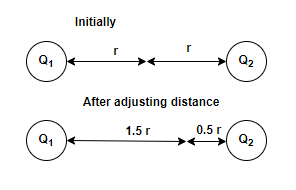# Problem: The electric potential at a point that is halfway between two identical charged particles is 300 V. What is the potential at a point that is 25% of the way from one particle to the other?

###### FREE Expert SolutionElectric potential is a scalar, therefore we'll sum the contribution of charges at the point of interest.

Initially:

$\begin{array}{rcl}\mathbf{V}& \mathbf{=}& \mathbf{k}\mathbf{\left(}\frac{{\mathbf{Q}}_{\mathbf{1}}}{\mathbf{r}}\mathbf{+}\frac{{\mathbf{Q}}_{\mathbf{2}}}{\mathbf{r}}\mathbf{\right)}\\ \mathbf{300}& \mathbf{=}& \mathbf{k}\mathbf{\left(}\frac{\mathbf{q}}{\mathbf{r}}\mathbf{+}\frac{\mathbf{q}}{\mathbf{r}}\mathbf{\right)}\\ \mathbf{300}& \mathbf{=}& \frac{\mathbf{2}\mathbf{kq}}{\mathbf{r}}\\ \mathbf{150}& \mathbf{=}& \frac{\mathbf{kq}}{\mathbf{r}}\end{array}$

94% (439 ratings)###### Problem Details

The electric potential at a point that is halfway between two identical charged particles is 300 V. What is the potential at a point that is 25% of the way from one particle to the other?